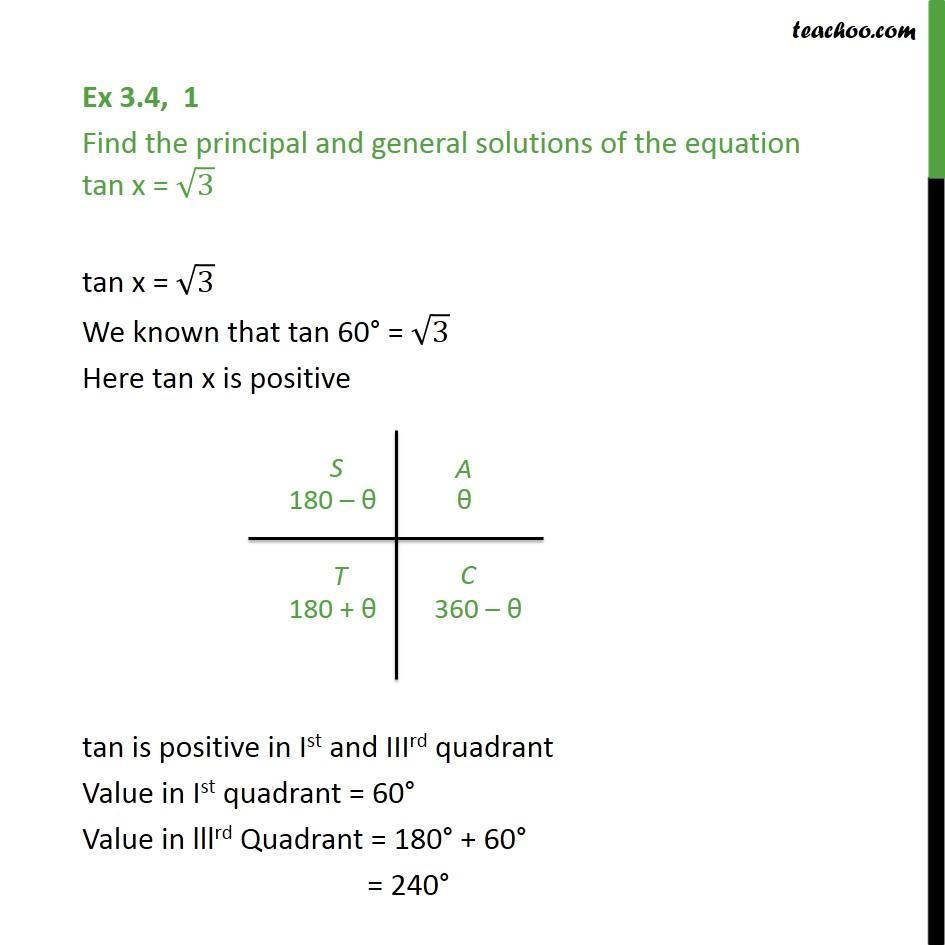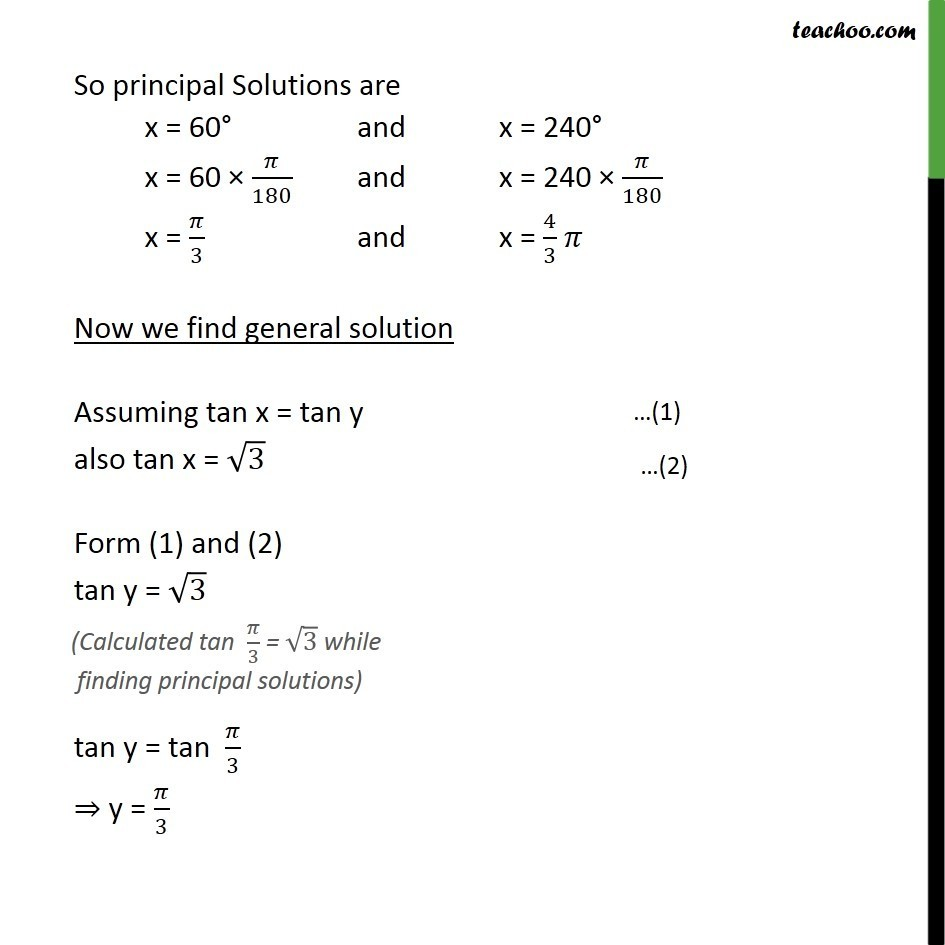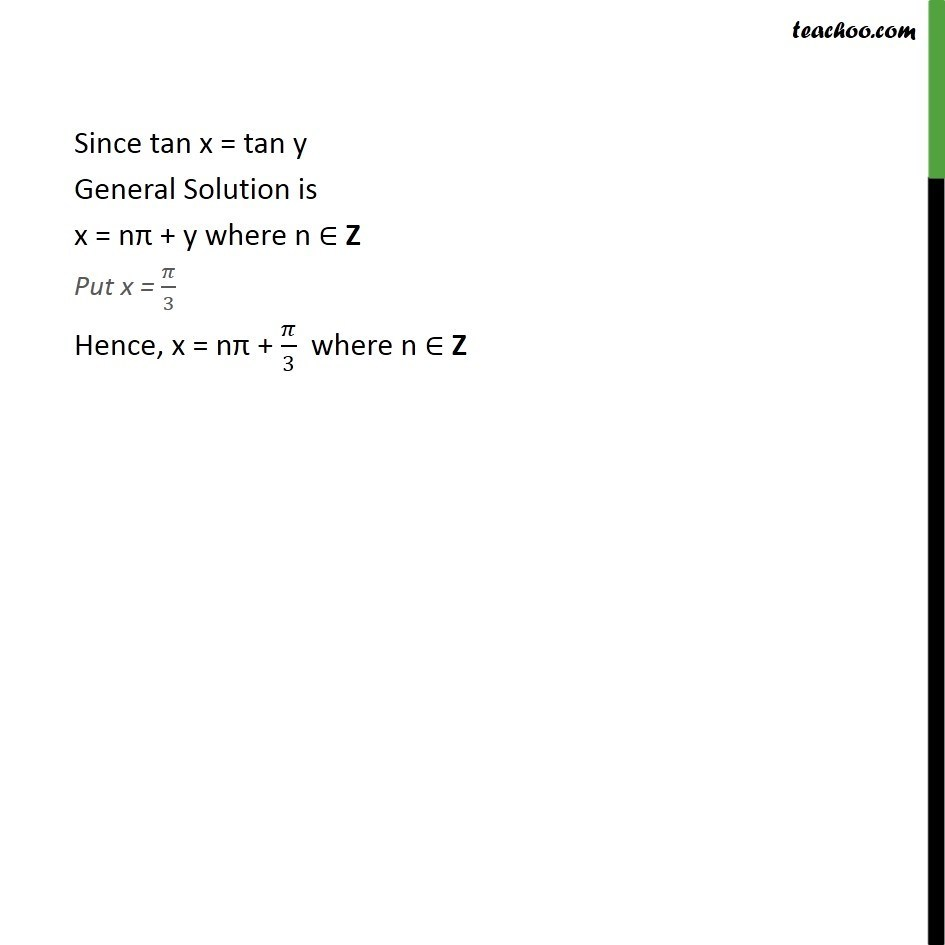1. Chapter 3 Class 11 Trigonometric Functions
2. Serial order wise
3. Ex 3.4

Transcript

Ex 3.4, 1 Find the principal and general solutions of the equation tan x = 3 tan x = 3 We known that tan 60 = 3 Here tan x is positive tan is positive in Ist and IIIrd quadrant Value in Ist quadrant = 60 Value in lllrd Quadrant = 180 + 60 = 240 So principal Solutions are x = 60 and x = 240 x = 60 /180 and x = 240 /180 x = /3 and x = 4/3 Now we find general solution Assuming tan x = tan y also tan x = 3 Form (1) and (2) tan y = 3 tan y = tan /3 y = /3 Since tan x = tan y General Solution is x = n + y where n Z Put x = /3 Hence, x = n + /3 where n Z

Ex 3.4